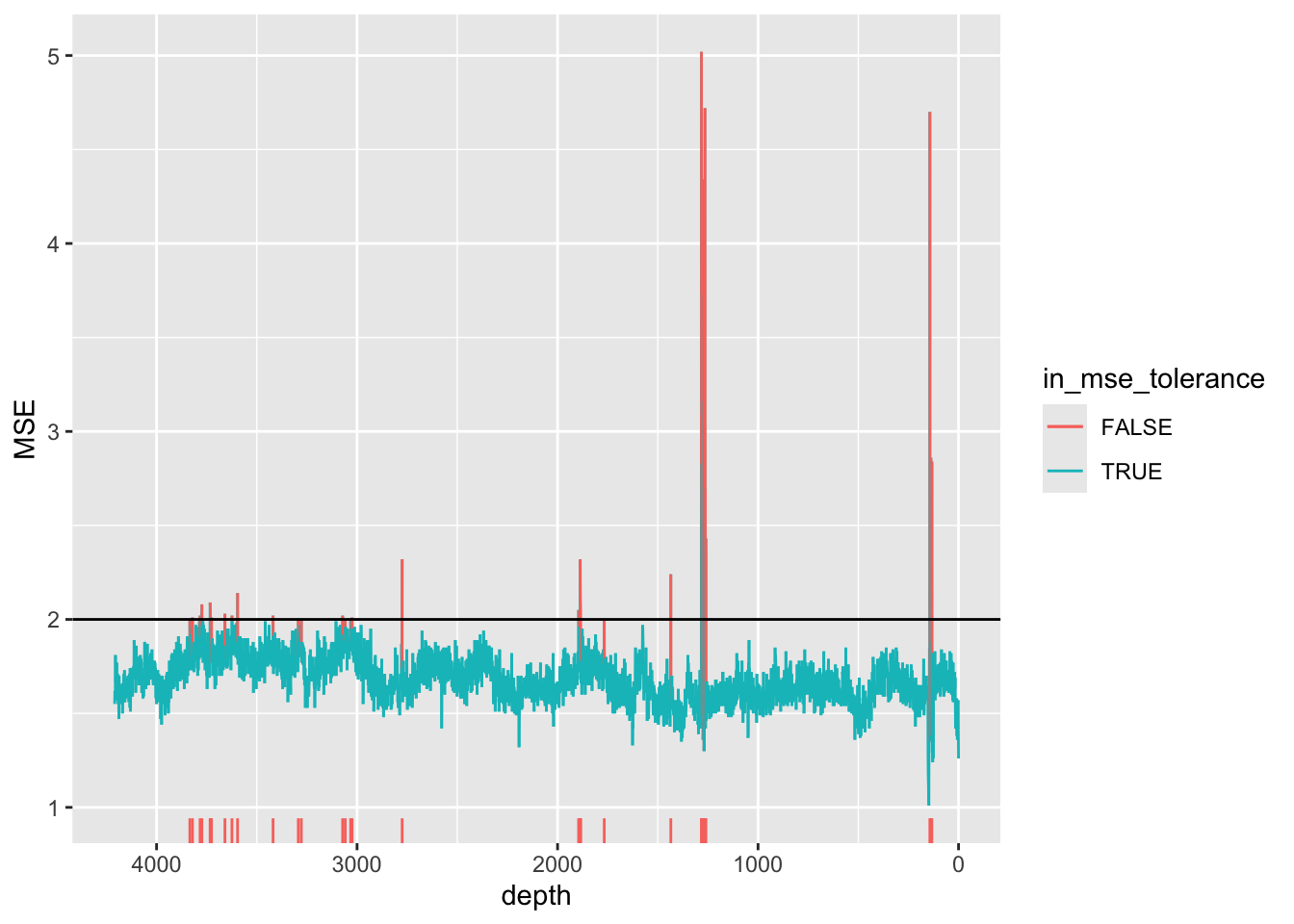## 3.2 Model Errors (MSE)

The mean-squared-error (MSE) of the model provides an indication of the goodness-of-fit for the peak fitting model implemented in the Q-Spec software. Operators aim to produce models with the lowest possible MSE, but compromises are sometimes required because normally the same model should be applied to observations that are being compared (for example, for the same core sequence).

``````CD166_19_xrf %>%
mutate(in_mse_tolerance = ifelse(MSE >=2, FALSE, TRUE)) %>%

ggplot(mapping = aes(x = depth,  y = MSE, col = in_mse_tolerance)) +
geom_line(aes(group = 1)) +
scale_x_reverse() +
geom_hline(yintercept = 2) +
geom_rug(sides = "b", data = . %>% filter(in_mse_tolerance == FALSE)) ``````